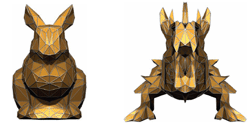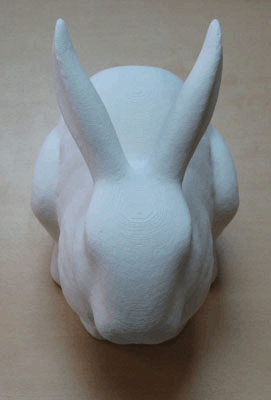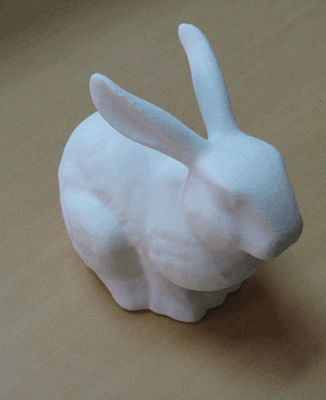Symmetrization
Niloy J. Mitra, Leonidas Guibas, Mark Pauly
ACM SIGGRAPH 2007

Abstract:

We present a symmetrization algorithm for geometric objects. Our algorithm enhances approximate symmetries of a model while minimally altering its shape. Symmetrizing deformations are formulated as an optimization process that couples the spatial domain with a transformation configuration space, where symmetries can be expressed more naturally and compactly as parametrized pointpair mappings. We derive closed-form solution for the optimal symmetry transformations, given a set of corresponding sample pairs. The resulting optimal displacement vectors are used to drive a constrained deformation model that pulls the shape towards symmetry. We show how our algorithm successfully symmetrizes both the geometry and the discretization of complex 2D and 3D shapes and discuss various applications of such symmetrizing deformations.

Result video:

3D printout of the Symmetrized Bunny:

For comparison, please check out the original Stanford bunny.Bibtex:

```@article{mgp_symmetrization_sig_07,
AUTHOR = "Niloy J. Mitra and Leonidas Guibas and Mark Pauly",
TITLE = "Symmetrization",
JOURNAL = "ACM Transactions on Graphics (SIGGRAPH)",
VOLUME = "26",
NUMBER = "3",
PAGES = "\#63, 1-8",
YEAR = "2007",
}```